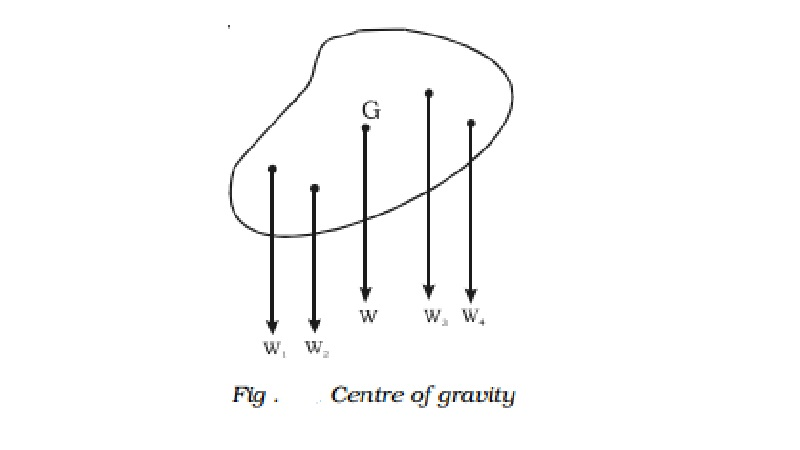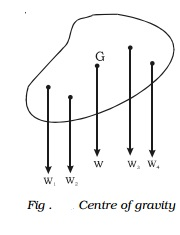Home | | Physics | Centre of gravity

# Centre of gravityA body may be considered to be made up of an indefinitely large number of particles, each of which is attracted towards the centre of the Earth by the force of gravity.

Centre of gravity

A body may be considered to be made up of an indefinitely large number of particles, each of which is attracted towards the centre of the Earth by the force of gravity. These forces constitute a system of like parallel forces. The resultant of these parallel forces known as the weight of the body always acts through a point, which is fixed relative to the body, whatever be the position of the body. This fixed point is called the centre of gravity of the body.The centre of gravity of a body is the point at which the resultant of the weights of all the particles of the body acts, whatever may be the orientation or position of the body provided that its size and shape remain unaltered.

In the Fig., W1,W2,W3,.. are the weights of the first, second, third, ... particles in the body respectively. If W is the resultant weight of all the particles then the point at which W acts is known as the centre of gravity. The total weight of the body may be supposed to act at its centre of gravity. Since the weights of the particles constituting a body are practically proportional to their masses when the body is outside the Earth and near its surface, the centre of mass of a body practically coincides with its centre of gravity.

Study Material, Lecturing Notes, Assignment, Reference, Wiki description explanation, brief detail
11th 12th std standard Class Physics sciense Higher secondary school College Notes : Centre of gravity |